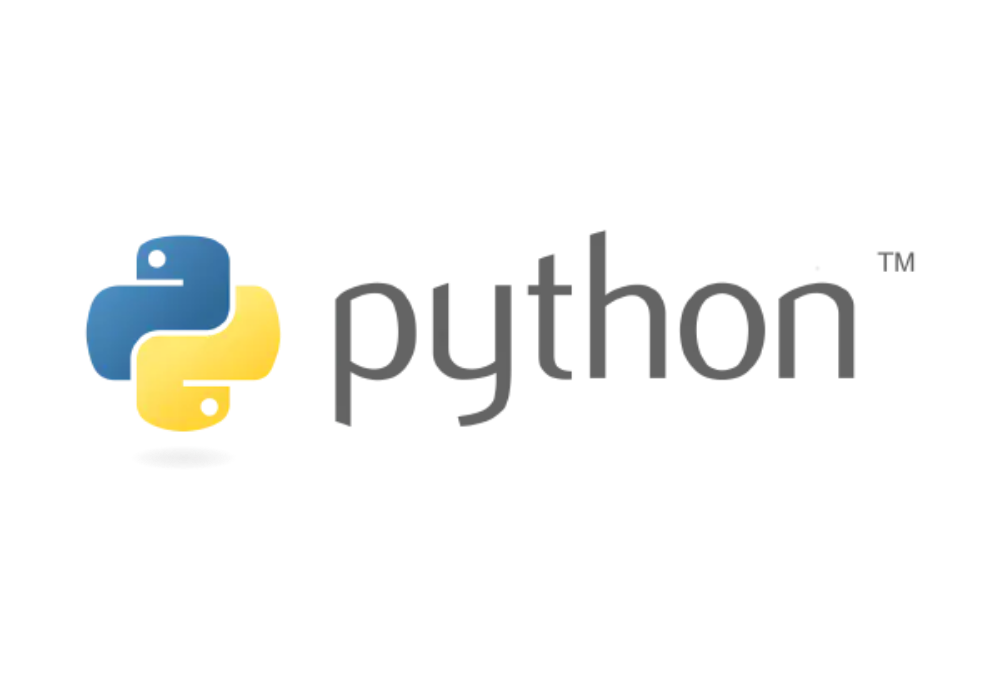Connect With Us:## Python101: 5. FunctionsPython
by Admin/ on 03 Jul 2021

## Python101: 5. Functions

Functions are organized, reusable segments of code used to implement a single, or associated, function, so I often say that functions are the basis for their use at programmer scale.

Functions can improve the modularity of applications, and code reuse. In the program design, often some common functional modules written as a function, placed in the function library for public choice. Good use of functions can reduce the workload of repeatedly writing program segments.

## How to define a function

There are several steps to define a function as follows

• The function code block starts with the def keyword followed by the function identifier name and parentheses ().
• Any incoming arguments and independent variables must be placed between the parentheses. Round brackets can be used between them to define parameters.
• The first line of a function may optionally use a document string - to hold the function description.
• The content of the function starts with a colon and is indented.
• `return` `[expression]` ends the function, optionally returning a value to the caller. A return without an expression is equivalent to returning None.

Syntax

``````def function name (list of arguments):
Function body
``````

By default, parameter values and parameter names are matched up in the order they are defined in the function declaration.

## Simple example

An example of the simplest function.

``````# Define a function
def hello() :
print("Hello World!")

# Call the function
hello()
``````

One more example of substitution of parameters.

``````# Define a function
def helloN(name) :
print("Hello World!", name)

# call function
helloN('neo')
``````

## Addition, subtraction, multiplication and division examples

We use functions to implement a basic addition, subtraction, multiplication and division operation.

``````# Define the function
return a+b

def reduce(a,b) :
return a-b

def multiply(a,b) :
return a*b

def divide(a,b) :
return a/b

# Call the function
print(reduce(12,2))
print(multiply(6,3))
print(divide(12,6))
``````

It was found that defining functions allows for multiple reuse of code.

## Multiple return values

In some cases, we need a function to return multiple values, and Python supports that as well.

``````# Define multiple return value functions
def more(x, y):
nx = x + 2
ny = y - 2
return nx, ny

# Call the function
x, y = more(10, 10)
print(x, y)
``````

## Recursive functions

Sometimes we need to call a function repeatedly to get a final value, and using recursive functions is the best solution.

Programming language, the function Func (Type a,……) directly or indirectly call the function itself, then the function is called recursive function. Recursive functions cannot be defined as inline functions

As an example, let’s calculate the factorial `n! = 1 x 2 x 3 x ... x n`, expressed as a function fact(n), it can be shown that

``````fact(n) = n! = 1 x 2 x 3 x ... x (n-1) x n = (n-1)! x n = fact(n-1) x n
``````

Therefore, `fact(n)` can be expressed as `n x fact(n-1)`, and only `n=1` requires special treatment.

Thus, `fact(n)` is written out recursively as

``````def fact(n):
if n==1:
return 1
return n * fact(n - 1)
``````

This completes the definition of a recursive function.

Let’s try to call what the factorial of 6 is, by calling

``````print(fact(6))
# Output content
# 720
``````

Similar needs can be implemented in this way.

## Summary

This section gives you an introduction to using Python functions.

Reference